﻿ 基于悬链线方程的海上横向补给高架索系统静力学分析
 舰船科学技术2016, Vol. 38Issue (3): 37-40PDF

Statics analysis of alongside-arranged highline cable replenishment system at sea based on catenary equation
GUO Fei, LI Jing-wei
Marine Design & Research Institute of China, Shanghai 200011, China
Abstract: Statics analysis model is established based on catenary equation for alongside-arranged highline cable replenishment system at sea after considering influence of cable's length and weight. Effects of several parameters, including cable's unit weight and tensile force, are then discussed. It is found when the ratio of horizontal tension to cargo weight exceeds 4, the deflection of highline cable is ignorable to safely transfer cargos.
Key words: replenishment at sea    highline cable    catenary equation    cable configuration analysis
0 引言

1 力学分析模型 1.1 基本假设

1）忽略船体在波浪中的运动；

2）假设高架索为质量均匀分布的柔性构件，仅受轴向拉伸作用，忽略其轴向弹性变形；

3）假设高架索水平张力恒定；

4）假设补给品在高架索上的运动缓慢平稳。

1.2 静力学分析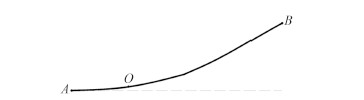图 1 悬挂点位于接收点之上时的线形 I Fig. 1 Cable configuration I when suspension point above receiving point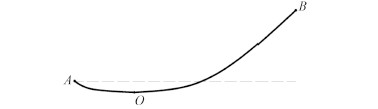图 2 悬挂点位于接收点之下时的线形 II Fig. 2 Cable configuration II when suspension point below receiving point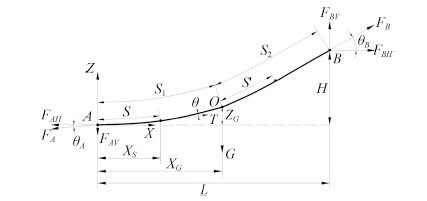图 3 高架索系统受力分析图 Fig. 3 Force analysis of the highline cable system

S1段内，定义原点为A的局部坐标系。取距点A水平距离为xs的一段高架索做力学分析，其长度为S

 $\sum{{{F}_{x}}}={{F}_{A}}\cos {{\theta }_{A}}=T\cos \theta \text{,}$ (1)
 $\sum{{{F}_{y}}=T\sin \theta -{{F}_{A}}\sin {{\theta }_{A}}-w\cdot S=0}.$ (2)

 ${{H}_{0}}\tan \theta -{{H}_{0}}\tan {{\theta }_{A}}-w\cdot S=0,$ (3)

 $\frac{\text{d}\left(\tan \theta \right)}{\sqrt{1+{{\tan }^{2}}\theta }}=\frac{w}{{{H}_{0}}}\text{d}x,$ (4)

 $\left\{ \begin{matrix} \theta =\arctan \left( sh\left( \frac{w}{{{H}_{0}}}{{x}_{s}}+{{C}_{1}} \right) \right), \\ \begin{array}{*{35}{l}} z=\frac{{{H}_{0}}}{w}ch\left( \frac{w}{{{H}_{0}}}{{x}_{s}}+{{C}_{1}} \right)+{{C}_{2}}, \\ S=\frac{{{H}_{0}}}{w}sh\left( \frac{w}{{{H}_{0}}}{{x}_{s}}+{{C}_{1}} \right)+{{C}_{3}}\text{.} \\ \end{array} \\ \end{matrix} \right.$ (5)

 \begin{align} & {{H}_{0}}sh\left( \frac{w}{{{H}_{0}}}{{X}_{G}}+ash\left( \frac{w{{Z}_{G}}}{2{{H}_{0}}}\cdot \frac{1}{sh\left( \frac{w{{X}_{G}}}{2{{H}_{0}}} \right)} \right)-\frac{w{{X}_{G}}}{2{{H}_{0}}} \right)+G= \\ & {{H}_{0}}sh\left( ash\left( \frac{w\left( H-{{Z}_{G}} \right)}{2{{H}_{0}}}\cdot \frac{1}{sh\left( \frac{w\left( L-{{X}_{G}} \right)}{2{{H}_{0}}} \right)} \right)-\frac{w\left( L-{{X}_{G}} \right)}{2{{H}_{0}}} \right), \\ \end{align} (6)

 \begin{align} & {{H}_{0}}sh\left( ash\left( \frac{w\left( H-{{Z}_{G}} \right)}{2{{H}_{0}}}\cdot \frac{1}{sh\left( \frac{w\left( L-{{X}_{G}} \right)}{2{{H}_{0}}} \right)} \right)-\frac{w\left( L-{{X}_{G}} \right)}{2{{H}_{0}}} \right)+ \\ & {{H}_{0}}sh\left( ash\left( \frac{-w{{Z}_{G}}}{2{{H}_{0}}}\cdot \frac{1}{sh\left( \frac{w{{X}_{G}}}{2{{H}_{0}}} \right)} \right)-\frac{w{{X}_{G}}}{2{{H}_{0}}} \right)=G. \\ \end{align} (7)

1.3 力学模型验证表 1 高架索模型主要参数 Tab.1 Main parameters of the highline cable model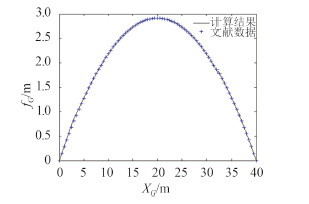图 4 悬挂点挠度曲线对比（H0 = 20 kN） Fig. 4 Comparison of deflection curve of suspension point（H0 = 20 kN）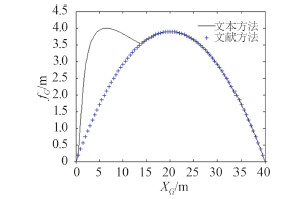图 5 悬挂点挠度曲线对比（H0 = 15 kN） Fig. 5 Comparison of deflection curve of suspension point（H0 = 15 kN）
2 高架索系统的线形分析

2.1 架索单位长度质量对线形的影响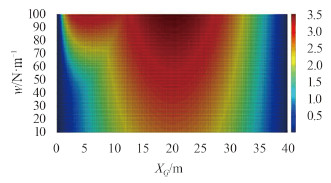图 6 不同架索自重时悬挂点挠度云图 Fig. 6 Deflection nephogram of suspension point when cable’s weight differs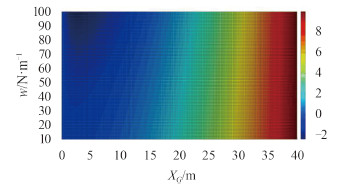图 7 不同架索自重时悬挂点垂向位置云图 Fig. 7 Vertical position nephogram of suspension point when cable’s weight differs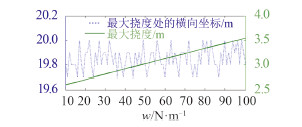图 8 不同架索自重时悬挂点最大挠度 Fig. 8 Maximal deflection of suspension point when cable’s weight differs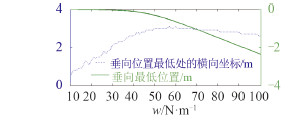图 9 不同架索自重时悬挂点最低位置 Fig. 9 Lowest position of suspension point when cable’s weight differs
2.2 架索水平张力对线形的影响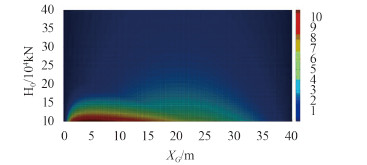图 10 不同水平张力时悬挂点挠度云图 Fig. 10 Deflection nephogram of suspension point when horizontal tensile force differs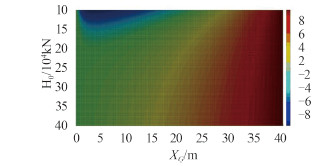图 11 不同水平张力时悬挂点挠度云图 Fig. 11 Vertical position nephogram of suspension point when cable’s weight differs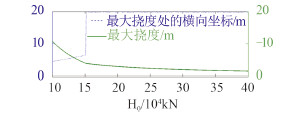图 12 不同水平张力时悬挂点最大挠度 Fig. 12 Maximal deflection of suspension point when horizontal tensile force differs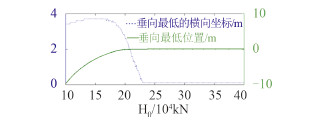图 13 不同水平张力时悬挂点最低位置 Fig. 13 Lowest position of suspension point when horizontal tensile force differs
3 结语

  严梅剑. 海上航行横向补给装置选型设计[J]. 船舶, 2004(1):51-53.  邓凯, 李红涛, 余建星. 高架索航行补给中船舶在波浪中的运动性能研究[J]. 船舶力学, 2009, 13(2):217-225.  赵小兵, 赵伟, 马良荔, 等. Ian航行横向补给高架索张力及张力波动控制参数仿真[J]. 舰船电子工程, 2007, 27(5):115-117.  何学军, 张良欣, 任爱娣. 海上横向补给系统高架索参数影响分析[J]. 海军工程大学学报, 2009, 21(5):52-56, 66.  马仁刚, 张良欣, 何学军. 横向干货补给系统高架索的静力学分析[J]. 舰船科学技术, 2012, 34(2):47-49, 80.  卢永锦. 海上横向补给高架索道系统数学模型研究[J]. 中国造船, 1996(1):19-22.  阎宏生, 余建星, 郭海涛. 海上轻型高架索补给系统的力学分析及安全性研究[J]. 海洋技术, 2006, 25(2):39-43.  余建星, 李红涛. 高架索海上补给装置在小型船舶补给上的应用[J]. 海洋技术, 2005, 24(2):59-62.  施平安, 何立居, 叶家玮. 两船波浪中并靠补给相对运动模型试验研究[J]. 船海工程, 2012, 41(5):26-30.